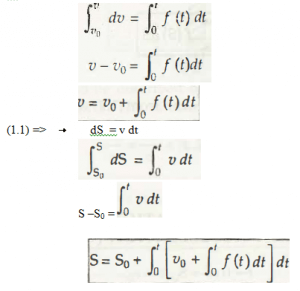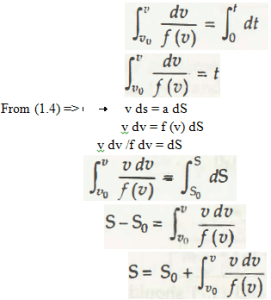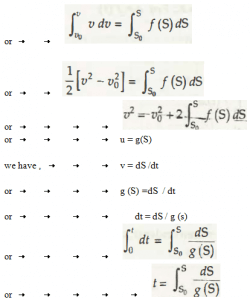In equation 1.5.1, a = f (t)

This states acceleration which is a function of time.

Taking from the equation (1.2),

dv / dt =a = f (t)

Or calculation for dv = f (t) dt

On integrating it, the calculated equation is,In equation 1.5.2, a = f (v)

This states acceleration which is a function of velocity.

Taking from the equation (1.3),

a = dv / dt = f (v)

Or calculation for dv / f (v) dt

On integrating it, the calculated equation is,In equation 1.5.3, a = f (S)

This states acceleration which is a function of distance.

Taking from the equation (1.4),

V dv = a dS

Or calculation for v dv = f (s) Ds

On integrating it, the calculated equation is,Links of Next Mechanical Engineering Topics:-### Customer Reviews

My Homework Help
Rated 5.0 out of 5 based on 510 customer reviews at